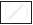Share

# Use the Sign of >, < Or = in the Box to Make the Statements True.23 – 41 + 11 and 23 – 41 – 11 - CBSE Class 7 - Mathematics

ConceptIntroduction of Integer

#### Question

Use the sign of >, < or = in the box to make the statements true.

23 – 41 + 1123 – 41 – 11

#### Solution

23 - 41 + 1123- 41 - 11

⇒ -7 >- 29

Is there an error in this question or solution?

#### APPEARS IN

NCERT Solution for Mathematics for Class 7 (2018 to Current)
Chapter 1: Integers
Ex. 1.10 | Q: 9.3 | Page no. 5
Solution Use the Sign of >, < Or = in the Box to Make the Statements True.23 – 41 + 11 and 23 – 41 – 11 Concept: Introduction of Integer.
S